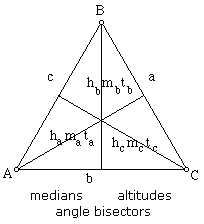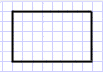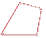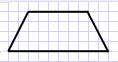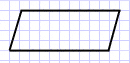Special Polygons:Triangle : A triangle is a three-sided polygon.     The midsegment of a triangle is equal to 1/2 the base and is pararellel to the base.      The three midsegments of a triangle devide the triangle into four congruent triangles. Rectangle : A quadrilateral with all angles as right angles.     The diagonals of rectangle are congruent.Square : A rectangle with all congruent sides.Kite :  A quadrilateral with exactly two pairs of distinct congruent consecutive sides.      The diagonals of a kite are perpendicular.       The diagonal connecting the vertex angles of a kite is the perpendicular bisector of the other diagonal.      The nonvertex angles of a kite are congruent.      A diagonal bisects the vertex angles of a kite.Trapezoid : A quadrilateral with exactly one pair of parallel sides.      Consecutive angeles between the bases of a trapezoid are supplementary.      An isosceles trapezoid is a trapezoid whose two non-parallel sides is the same length.      The base angles of an isoceles triangle are congruent.      The diagonals of an isosceles trapezoid are congruent.      The midsegment of a trapezoid is equal in length to the average of the two bases and is parallel to the base.Parallelogram : A quadrilateral whose opposite sides are parallel.      The opposite sides of a parallelogram are congruent.      Consecutive angles in a parallelogram are supplementary.      Opposite angles in a parallelogram are congruent.       The diagonals of a parallelogram bisect each other.Rhombus : A parallelogram with all four sides congruent.      The diagonals of a rhombus are perpendicular bisectors of each other.       The diagonals of a rhombus bisect the angles of the rhombus.Here is a Venn Diagram to help catagorize the different Quadrilateral:* An apothem is the perpendicular distance from the center of a polygon's circumscribed circle to one of it's sides.